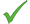Moduli spaces of algebraic curves and mathematical physics

••Applications accepted all year round
•Competition Funded PhD Project (Students Worldwide)

Project Description

The moduli space of curves is the space of all complex structures on a given compact two-dimensional surface. This is a complicated topological space with a very rich and interesting geometry. The moduli space of curves plays a central role in modern curve counting theories, like Gromov-Witten theory, which studies the number of algebraic curves with certain properties containing in some algebraic variety. Remarkably, the moduli space of curves also lies at the crossroad of many other mathematical disciplines, including the theory of integrable partial differential equations, differential geometry, singularity theory, combinatorics.

There are many starting points for a PhD project in this area. One can study the topological properties of the moduli space of curves, in particular, its cohomology groups. Another possibility could be to study the relations of the moduli space of curves with the theory of integrable partial differential equations. Certain differential geometric structures, related to the moduli space of curves, in particular, the Frobenius manifolds and their generalizations, could also be an object of research for a PhD project.

How good is research at University of Leeds in Mathematical Sciences?

FTE Category A staff submitted: 53.00

Research output data provided by the Research Excellence Framework (REF)

Click here to see the results for all UK universities

Email Now

Insert previous message below for editing?
You haven’t included a message. Providing a specific message means universities will take your enquiry more seriously and helps them provide the information you need.
Why not add a message here
* required field
Send a copy to me for my own records.